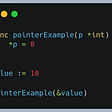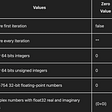# Problem

`Given an integer array, find if an integer p exists in the array such that the number of integers greater than p in the array equals to p.If such an integer is found return 1 else return -1.`

# Solving Process

## Simplify Input

This problem is not very difficult but to solve it we have to apply a well known technique to simplify the given inputs.

Let’s take a small example:

`2, 6, 1, 3`

This input should return 1 as 2 is a noble integer. We know that by counting the number of integers greater than 2 (3 and 4).

Yet, how to solve this problem without having an implementation in O(n²)? The answer is to simplify the inputs.

What if in our case the array was sorted?

`1, 2, 3, 6`

The integer 2 is at index 1, whereas the array size is 4. The success condition is the following:

`size(array) - index - 1 == array[i]`

Once the problem is solved using a simplification, we need to check the implications in terms of complexity. If our solution is acceptable, we generalize to the initial problem.

In our case, we have to:

• Sort the array => O(n log(n))
• Iterate over each element and check the previous condition => O(n)

It means the solution is O(n log(n)).

Bear in mind, sorting an array can’t be done with a better solution than a O(n log(n)) (like a merge sort for example).

Also, we have to make sure our solution covers all corner cases.

What about the following example, after being sorted:

`1, 2, 2, 3   x`

At index 1, the condition is going to be true. Yet, this should not be a match.

In our case, we also have to cover duplicate by checking if the next integer is equals to the current one.

A possible implementation in Java:

--

--

--

## More from solvingalgo

A Collection of Helpful Programming Resources

## Build A Chatbot with Microsoft Bot Framework Composer & Composite Entities## How to Start and Stop EC2 Instances Using AWS Lambda and CloudFormation## How to add Step Descriptions in Allure Reports (Gradle + Java)## Search Element in Row-wise and Column-wise Sorted Matrix## Managed Kubernetes Cluster (HA) for Side Projects## Allocations Crodo.io## Best Terraform Tutorial Guides: An Overview## Teiva Harsanyi

Software Engineer, Go, Rust, Java | 改善

## Go — pointers (basics)## Find Palindrome in a Sentence with Punctuation and/or Spaces in Golang## Object-Oriented Programming in Kotlin## Types in Go